## Scales are used to measure mass, length, capacity, and angles.

Most modern measuring devices that we use today are electronic and have digital displays, but your child needs to be able to read measurements that are analogue.

Examples are thermometers, weighing scales, spring balances, rulers, tape measures, measuring jugs and clocks.

A scale is a series of lines and numbers with a device that points to the measurement. If the pointer lines up exactly with a number, then it is easy to read off the number indicated. If however the pointer lines up between numbers then you have to understand what the divisions represent. Make sure that you use the correct units. mm, cm, m, kg, g etc.

It is important to know what unit of measurement is being used - the units are always labelled on the scale.

Before you start measuring - work out what each division on the scale is worth. For instance, if there are 10 divisions between numbers then each division is worth 1/1oth of the number - if there are only four divisions then they will be worth ¼, ½ and ¾. A common number of divisions is 5, so the divisions are worth 0.2, 0.4, 0.6 and 0.8.

To measure length we usually use a ruler or tape measure. A common question asks you to measure the lengths of different lines accurately.

Make sure that the zero mark on the ruler is line up with the beginning of the line - there is usually a blank section at the ends of the ruler to protect the scale section from damage.To measure the length of the line AB, line up the zero mark on point A and read off the point on the ruler at point B. .... 11 cm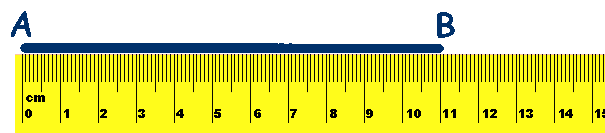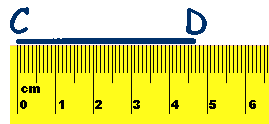To measure the length of the line CD, line up the zero mark on point C and read off the point on the ruler at point D. .... 4.7 cm

###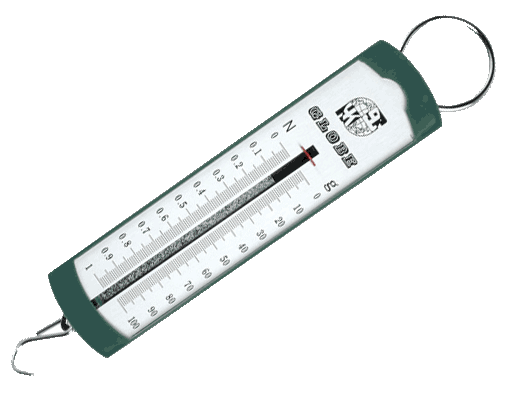Weighing scales often have a rotary dial to indicate the mass of an object.

##70 g or 2.5 oz

In school, Force meters (spring balances) are often used to measure the mass of an object. The scales use both grams and Newtons.

1 Newton = 100 grams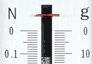Measuring jugs can be used to measure capacity of liquids.

Make sure that the jug or beaker is placed on a level surface.

You can also weigh objects to find their capacity.
First weigh the object when it is empty and then fill it with water and weigh it again. Subtract the empty weight from the full weight and you have the weight of the water. As 1 litre weighs 1 kg and 1 ml = 1 g
you can easily convert mass to capacity. 250 g = 250 ml.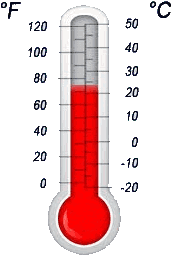Temperature is measured using a thermometer.

Temperature is measured in degrees Celsius (°C)

Water freezes at 0 °C

Water boils at 100 °C

### We measure angles with a protractor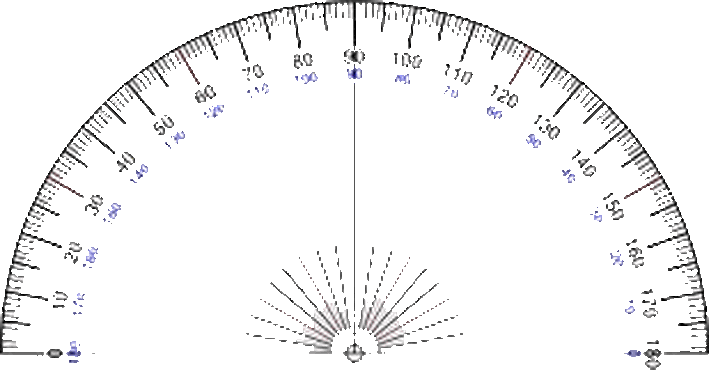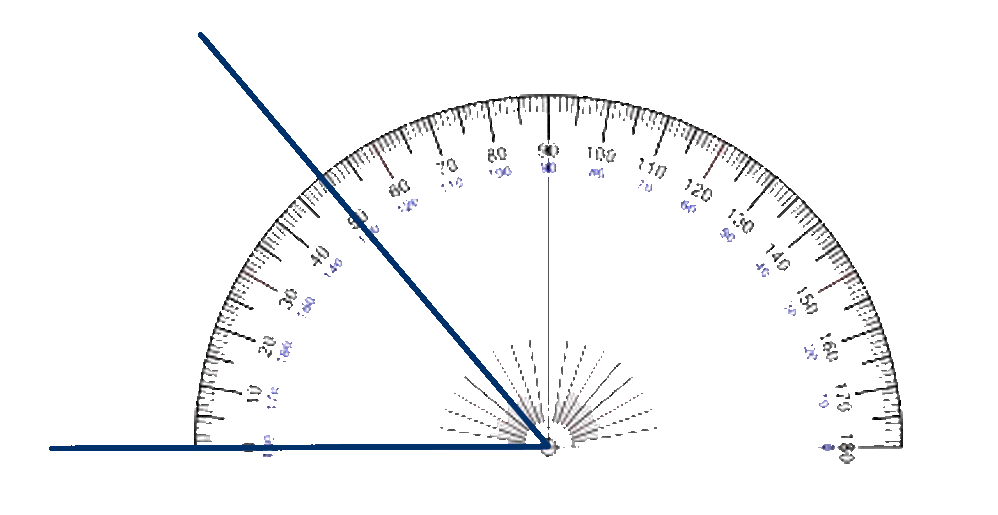To use a protractor, you position the protractor over the angle to be measured with the centre lined up exactly over the point of the angle and the zero line exactly over one line of the angle. Then look at where the other line of the angle intersects with the protractor and read off the angle - in this case 50°

## Identify the units and the value of the divisions on the scale.

### Play in Full Screen

Practice measuring with these worksheets
from Primary Resources